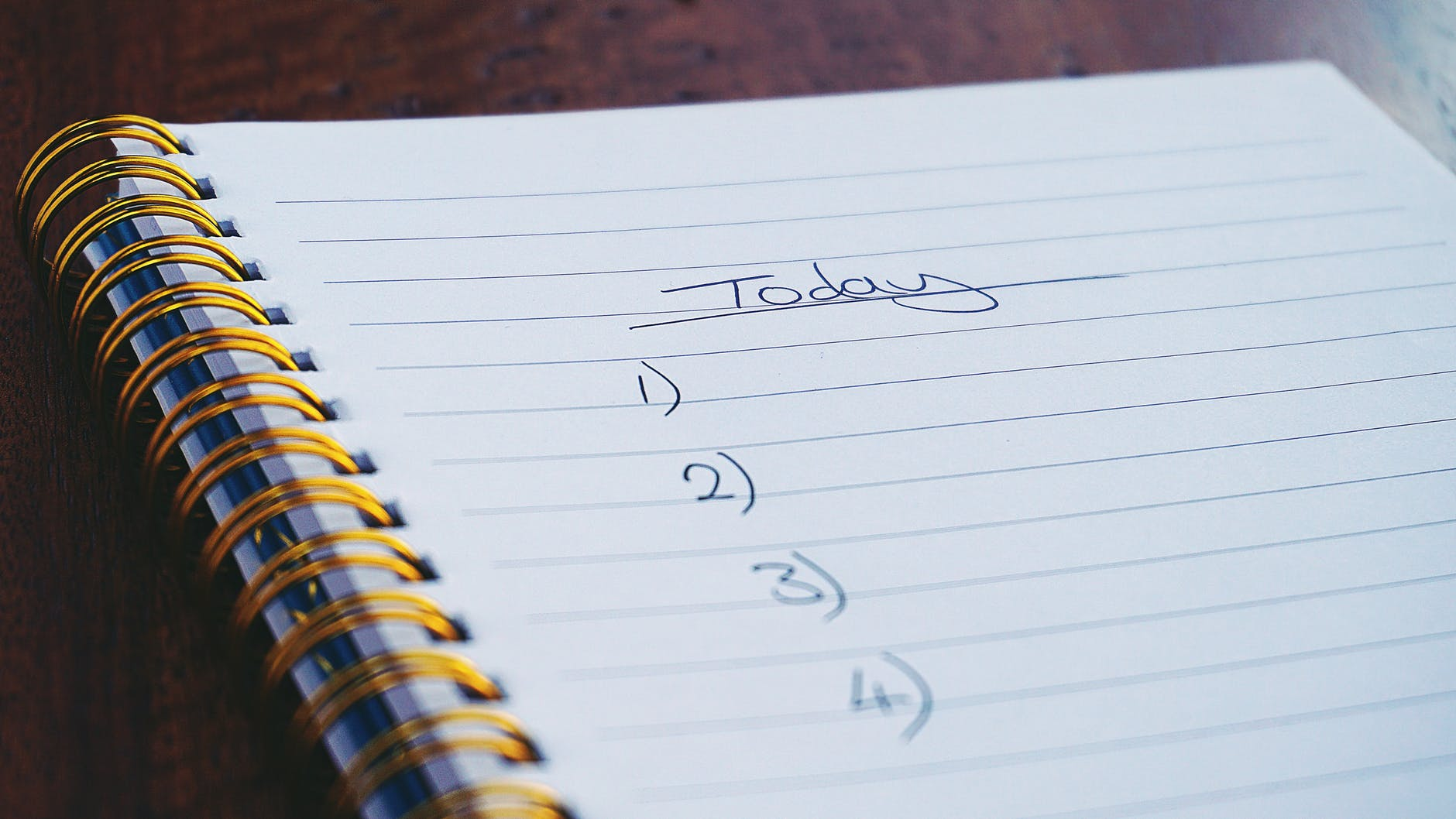# Lists in Python

Lists allow you to organize information. In the real world, we make list all the time to keep track of things. This same concept applies in Python when making list. A list is a sequence of stored data. By sequence, it is mean a data structure that allows multiple items to exist in a single storage unit. By making list we are explaining to the computer how to store the data in the computer’s memory.In this post, we learn the following about list

• How to make a list
• Accessing items in a list
• Looping through a list
• Modifying a list

Making a List

Making a list is not difficult at all. To make one you first create a variable name followed by the equal sign and then place your content inside square brackets. Below is an example of two different lists.

```numList=[1,2,3,4,5]
alphaList=['a','b','c','d','e']
print(numList,alphaList)
[1, 2, 3, 4, 5] ['a', 'b', 'c', 'd', 'e']```

Above we made two lists, a numeric and a character list. We then printed both. In general, you want your list to have similar items such as all numbers or all characters. This makes it easier to recall what is in them then if you mixed them. However, Python can handle mixed list as well.

Access a List

To access individual items in a list is the same as for a sting. Just employ brackets with the index that you want. Below are some examples.

```numList=[1,2,3,4,5]
alphaList=['a','b','c','d','e']

numList
Out: 1

numList[0:3]
Out: [1, 2, 3]

alphaList
Out: 'a'

alphaList[0:3]
Out: ['a', 'b', 'c']```

numList gives us the first value in the list. numList[0:3] gives us the first three values. This is repeated with the alphaList as well.

Looping through a List

A list can be looped through as well. Below is a simple example.

```for item in numList :
print(item)

for item in alphaList :
print(item)

1
2
3
4
5
a
b
c
d
e```

By making the two for loops above we are able to print all of the items inside each list.

Modifying List

There are several functions for modifying lists. Below are a few

The append() function as a new item to the list

```numList.append(9)
print(numList)
alphaList.append('h')
print(alphaList)

[1, 2, 3, 4, 5, 9]
['a', 'b', 'c', 'd', 'e', 'h']```

You can see our lists new have one new member each at the end.

You can also remove the last member of a list with the pop() function.

```numList.pop()
print(numList)
alphaList.pop()
print(alphaList)
[1, 2, 3, 4, 5]
['a', 'b', 'c', 'd', 'e']```

By using the pop() function we have returned our lists back to there original size.

Another trick is to merge lists together with the extend() function. For this, we will merge the same list with its self. This will cause the list to have duplicates of all of its original values.

```numList.extend(numList)
print(numList)
alphaList.extend(alphaList)
print(alphaList)

[1, 2, 3, 4, 5, 1, 2, 3, 4, 5]
['a', 'b', 'c', 'd', 'e', 'a', 'b', 'c', 'd', 'e']```

All the values in each list have been duplicated. Finally, you can sort a list using the sort() function.

```numList.sort()
print(numList)
alphaList.sort()
print(alphaList)
[1, 1, 2, 2, 3, 3, 4, 4, 5, 5]
['a', 'a', 'b', 'b', 'c', 'c', 'd', 'd', 'e', 'e']```

Now all the numbers and letters are sorted.

Conclusion

THere is way more that could be done with lists. However, the purpose here was just to cover some of the basic ways that list can be used in Python.